# Transistor Symbols

Transistor schematic symbols of electronic circuit - NPN, PNP, Darlington, JFET-N, JFET-P, NMOS, PMOS.

## Table of transistor symbols

 Symbol Name Description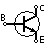NPN Bipolar Transistor Allows current flow when high potential at base (middle)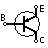PNP Bipolar Transistor Allows current flow when low potential at base (middle)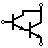Darlington Transistor Made from 2 bipolar transistors. Has total gain of the product of each gain.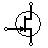JFET-N Transistor N-channel field effect transistor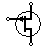JFET-P Transistor P-channel field effect transistor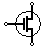NMOS Transistor N-channel MOSFET transistor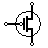PMOS Transistor P-channel MOSFET transistor

Here are the schematic symbols for some common transistor types:

1. NPN transistor symbol:
• The NPN transistor symbol consists of a triangle representing the emitter, a circle representing the collector, and a rectangle representing the base. The arrow in the symbol points from the emitter to the collector, indicating the direction of current flow through the transistor.
1. PNP transistor symbol:
• The PNP transistor symbol is similar to that of an NPN transistor, but with the arrow pointing in the opposite direction.
1. Darlington transistor symbol:
• The Darlington transistor symbol consists of two NPN transistors connected in series, with a circle representing the common collector and two rectangles representing the bases of the transistors. The arrow in the symbol points from the emitter to the collector, indicating the direction of current flow through the transistor.
1. JFET-N (Junction Field-Effect Transistor - N-channel) symbol:
• The JFET-N symbol consists of a triangle representing the drain, a rectangle representing the gate, and a line representing the source. The arrow in the symbol points from the source to the drain, indicating the direction of current flow through the transistor.
1. JFET-P (Junction Field-Effect Transistor - P-channel) symbol:
• The JFET-P symbol is similar to that of a JFET-N, but with the arrow pointing in the opposite direction.
1. NMOS (N-channel MOSFET) symbol:
• The NMOS symbol consists of a triangle representing the drain, a rectangle representing the gate, and a line representing the source. The arrow in the symbol points from the source to the drain, indicating the direction of current flow through the transistor.
1. PMOS (P-channel MOSFET) symbol:
• The PMOS symbol is similar to that of an NMOS, but with the arrow pointing in the opposite direction.

It is important to note that the direction of the arrow in the transistor symbol indicates the direction of current flow through the transistor, and not the direction of the voltage drop across the transistor.

Electronic symbols ►